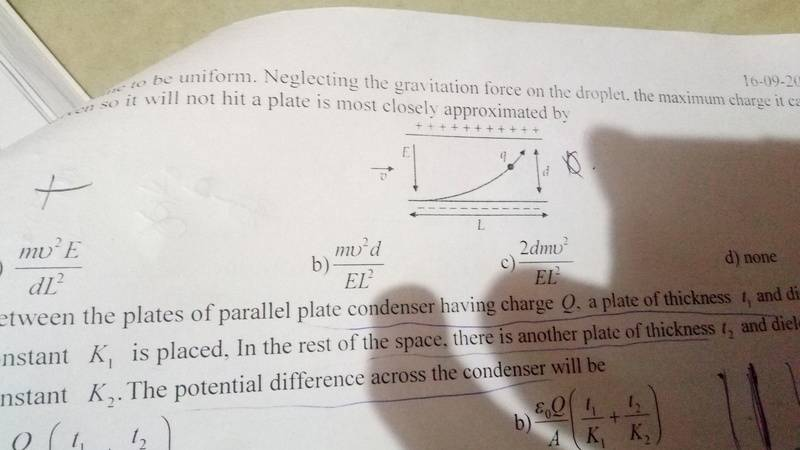# Charge entering into the field between capacitor plates

## Homework Statement

A negative charge of mass m enters into the field between two deflecting parallel plates with length 'l' separated by distance 'd' with a velocity 'v'.Assuming electric field to be uniform,find the maximum charge so that it will not hit a plate

## Homework Equations

Kinematic and electrostatic equations[/B]

## The Attempt at a Solution

F=qE
Work done=qEd
qEd=1/2mv^2
q=mv^2/Ed

I am not sure this is right and that is why i require help

Delta2
Homework Helper
Gold Member
What is the angle of the velocity with the electric field vector? Is there a figure provided it with this? Can you upload the figure/image?
If it is as I suspect, that the velocity is initially perpendicular to the e-field, then I am afraid using the work energy theorem will get you nowhere. You need to use kinematics equations for projectile motion , with vertical acceleration ##g-\frac{Eq}{m}##, initial velocity ##v_{0x}=v## ,and ##\theta=0##.

What is the angle of the velocity with the electric field vector? Is there a figure provided it with this? Can you upload the figure/image?
If it is as I suspect, that the velocity is initially perpendicular to the e-field, then I am afraid using the work energy theorem will get you nowhere. You need to use kinematics equations for projectile motion , with vertical acceleration ##g-\frac{Eq}{m}##, initial velocity ##v_{0x}=v## ,and ##\theta=0##.

https://ibb.co/bDJxReCan you elaborate on why WE theorem cannot be used?
Note:gravity can be neglected

#### Attachments

Last edited by a moderator:
Delta2
Homework Helper
Gold Member
Ok I see now we neglect the gravitational force, so our acceleration is just ##a=\frac{Eq}{m} (1)##. Indeed v is perpendicular to e-field and the charge enters exactly at the bottom of the plates.
The exercise asks you the maximum charge so that it doesn't hit a plate, how work energy theorem could possibly help us with what the exercise asks? There is no way to use what the exercise ask us (not to hit a plate) together with WE theorem.
Instead use kinematics equations and determine what the charge should be so that
$$vt_0=L$$ , $$d\leq\frac{1}{2}at_0^2$$

Solve the first for ##t_0## and replace it in the second equation. Also use equation (1)

Last edited:
Delta2
Homework Helper
Gold Member

WE theorem is about initial and final velocities and work done. The problem here deals with conditions on the spatial coordinates of the particle, those spatial coordinates appear nowhere in the WE theorem, that's why it cant be used here.

WE theorem is about initial and final velocities and work done. The problem here deals with conditions on the spatial coordinates of the particle, those spatial coordinates appear nowhere in the WE theorem, that's why it cant be used here.
Cool. But which kinematic equation do i exactly use here?
I am ending up with more than one variable to solve.

Delta2
Homework Helper
Gold Member
Cool. But which kinematic equation do i exactly use here?
I am ending up with more than one variable to solve.
read again post #4 I edited it abit

read again post #4 I edited it abit

What is the use of L in this equation?

And is the final velocity of the charge 0?

Delta2
Homework Helper
Gold Member
No the velocity of the charge is not 0 when it exits the field.
In order for the particle not to hit the plate, its movement must be such so that when it has cover L distance in the x-axis and thus ready to exit the field, the distance covered in the y-axis must be smaller than d. Or more simply, when its x-coordinate becomes L, its y-coordinate must be smaller than d.

rude man
Homework Helper
Gold Member
Analogy: a mass is thrown horizontally from a platform d meters above the ground. How far will it go horizontally before it hits the ground?
Thing here is you can't use g = 9.81 m/sec2, you need to come up with the equivalent of g due to electrostatic as opposed to gravitational attraction.